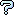All about flooble | fun stuff | Get a free chatterbox | Free JavaScript | Avatarsperplexus dot infoBasic Factoring (Posted on 2007-03-21)When math students are taught factoring, usually the first thing they learn are special factoring identities such as:

1: a˛-b˛= (a-b)(a+b)
2: ał-bł = (a-b)(a˛+ab+b˛)
3: ał+bł = (a+b)(a˛-ab+b˛)

Find a factorization of the expression x4+x2+1 using only those identities.

 See The Solution Submitted by Brian Smith Rating: 3.0000 (1 votes)Comments: ( Back to comment list | You must be logged in to post comments.)How's this? (Probable spoiler)| Comment 1 of 4

x^4 + x˛ + 1
x^4 - x˛ + 2x˛ + x - x + 1  [add zero as -x˛ + x˛ + x - x]
(x˛ + x)(x˛ - x) + x˛ + x + x˛ - x + 1  [rule 1]
(x˛ + x)(x˛ - x) + (x˛ + x) + (x˛ - x + 1) [group terms]
(x˛ + x)(x˛ - x + 1) + (x˛ - x + 1) [monomial factor]
(x˛ + x +1)(x˛ - x + 1) [monomial factor]

I assume this is ok as the factoring either involves rule 1 or does not involve an identity.

 Posted by Jer on 2007-03-21 11:07:37Please log in:

 Search: Search body:
Forums (1)